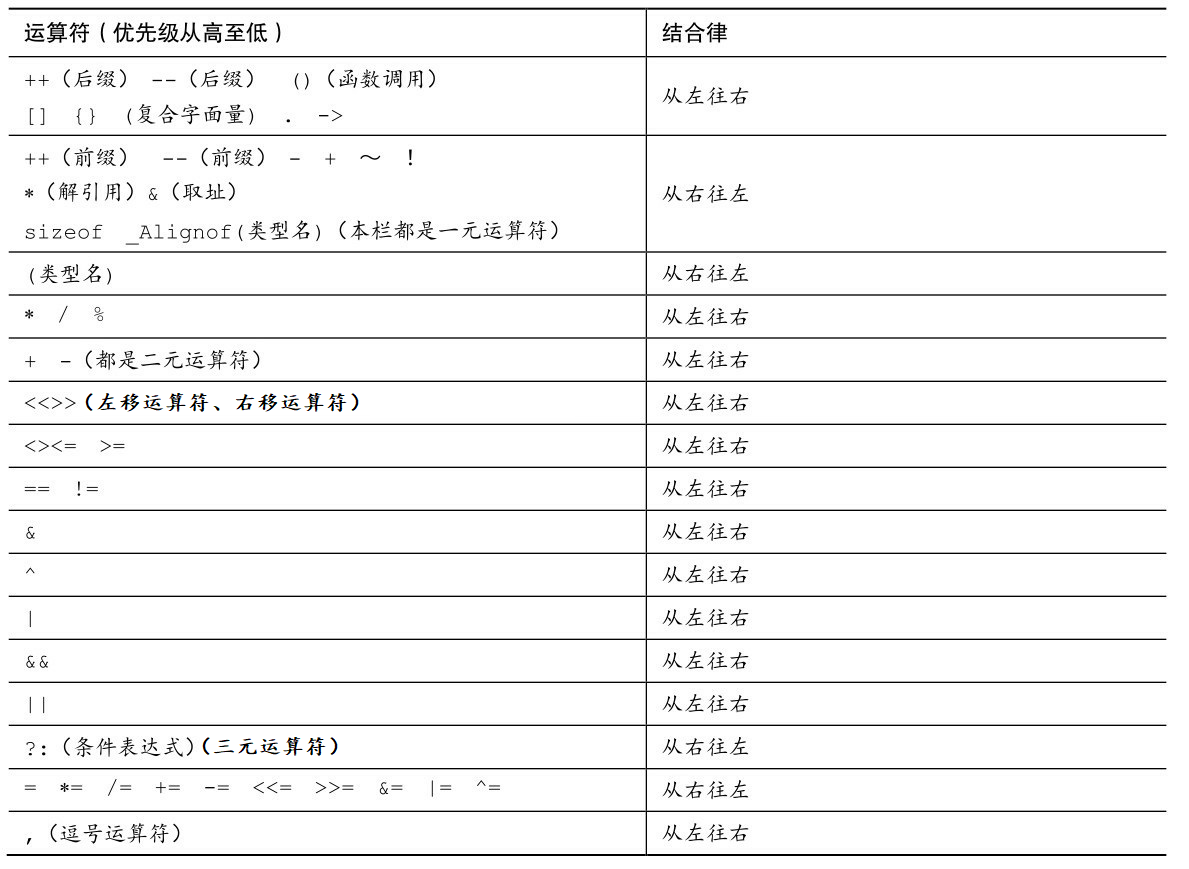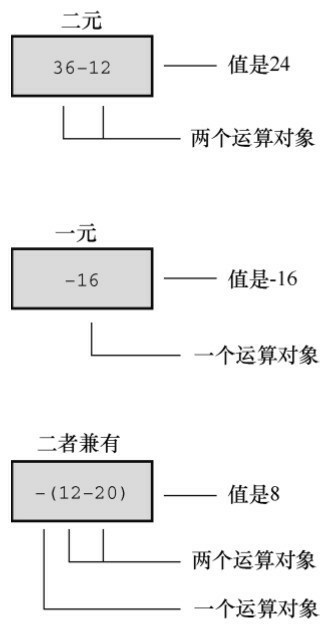`算术运算符` 用于处理四则运算
`赋值运算符` 用于将表达式的值赋给变量
`比较运算符` 用于表达式的比较，并返回一个真值或假值
`逻辑运算符` 用于根据表达式的值返回真值或假值
`位运算符` 用于处理数据的位运算
`sizeof 运算符` 用于求字节数长度

# 1. 算术运算符

`+` 正号 +3 3
`-` 负号 -3 -3
`+` 10 + 5 15
`-` 10 - 5 5
`*` 10 * 5 50
`/` 10 / 5 2
`%` 取模(取余) 10 % 3 1
`++` 前自增 a=2; b=++a; a=3; b=3;
`++` 后自增 a=2; b=a++; a=3; b=2;
`--` 前自减 a=2; b=–a; a=1; b=1;
`--` 后自减 a=2; b=a–; a=1; b=2;

• 取余（`%`）：被除数和除数进行除法运算，舍弃商保留其余数。
• 前缀自增（`++`）：运算符`++`写到变量前面，先进行自增运算，然后取变量的值
• 后缀自增（`++`）：运算符`++`写到变量后面，先取变量的值，然后进行自增运算
• 前缀自减（`--`）：运算符`--`写到变量前面，先进行自增运算，然后取变量的值
• 后缀自减（`--`）：运算符`--`写到变量后面，先取变量的值，然后进行自减运算1. 对于变量自身来说，不论是`前自增/自减`还是`后自增/自减`，最后变量的值都会增/减 1，这种情况下前/后自增、前/后自减是等价的
2. 如果想要取出某个自增/自减变量的值:
• 后自增/自减是先取变量的值，然后变量再进行自增/自减运算
• 前自增/自减是变量先进行自增/自减运算，然后再取该变量的值。

# 2. 赋值运算符

`=` 赋值 a=2; b=3; a=2; b=3;
`+=` 加等于 a=0; a+=2;（a=a+2） a=2;
`-=` 减等于 a=5; a-=3;（a=a-3） a=2;
`*=` 乘等于 a=2; a*=2;（a=a*2） a=4;
`/=` 除等于 a=4; a/=2;（a=a/2） a=2;
`%=` 模等于 a=3; a%2;（a=a%2） a=1;

== 相等于 4 == 3 0
!= 不等于 4 != 3 1
< 小于 4 < 3 0
> 大于 4 > 3 1
<= 小于等于 4 <= 3 0
>= 大于等于 4 >= 1 1

# 4. 逻辑运算符

`!` !a 如果a为假，则!a为真；如果a为真，则!a为假。
`&&` a && b 如果a和b都为真，则结果为真，否则为假。
|| a || b 如果a和b有一个为真，则结果为真，二者都为假时，结果为假。
1. 逻辑与（&&）：当所有条件都为 true 时，返回 true，否则返回 false。
2. 逻辑或（||）：当至少一个条件为 true 时，返回 true，否则返回 false。
3. 逻辑非（!）：对一个条件取反。
• 如果条件为true，则返回 false
• 如果条件为false，则返回 true。

# 5. 位运算符

• `按位与运算符：&`
• `按位或运算符：|`
• `按位异或运算符：^`
• `按位取反运算符：~`
• `左移运算符：<<`
• `右移运算符：>>`

## 5.1 按位与

• 整形数 2 对应的二进制数是`10`
• 整形数 4 对应的二进制数是`100`

## 5.2 按位或

• 整形数 2 对应的二进制数是`10`
• 整形数 4 对应的二进制数是`100`

## 5.3 按位异或

### 5.3.1 基本使用

• 整形数 2 对应的二进制数是`10`
• 整形数 4 对应的二进制数是`110`

### 5.3.2 实际应用

1. 交换两个变量的值：通过按位异或可以交换两个变量的值，而不需要使用临时变量。示例：

2. 加密与解密：按位异或可用于简单的加密和解密算法。通过将数据与一个密钥按位异或，可以对数据进行加密。同样地，将加密后的数据再与密钥按位异或，可以解密数据。示例：

## 5.4 左移和右移

• 对于有符号数，`使用算术右移`，用符号位填充左侧空位
• 对于无符号数，用 0 填充左侧空位

• 对于正数，右移一位相当于除以 2，向左移一位相当于乘以 2

• 在右移操作中，负数右移一位并不严格等于除以2，而是依赖于具体的编译器实现方式，因为 C 语言中没有明确规定。一种常见的实现方式是使用算术右移，即保留符号位并用符号位填充左侧空出的位。因此，负数右移一位并不一定等于除以2。

• 对于除以2的操作，除非对被除数是无符号整数，否则建议使用除法运算符（/）来达到目的。

# 6. 运算符优先级• `一元运算符，即单目运算符`
• `二元运算符，即双目运算符`
• `三元运算符，即三目运算符`